# Kerala Syllabus 10th Standard Chemistry Solutions Chapter 5 Compounds of Non-Metals

## Kerala State Syllabus 10th Standard Chemistry Solutions Chapter 5 Compounds of Non-Metals

### Compounds of Non-Metals Text Book Questions and Answers

Text Book Page No: 79

Sslc Chemistry Chapter 5 Kerala Syllabus  Question 1.
Take a little ammonium chloride (NH4Cl) in a watch glass and add a little calcium hydroxide (Ca(OH)2) to it. Stir well. Can you sense any smell?
Irritating smell is experienced.

Compounds Of Non Metals Class 10 Kerala Syllabus Question 2.
Show wet blue and red litmus papers over the watch glass one by one. Which litmus paper shows a color change ?
Red litmus turns to blue

5 nonmetals Question 3.
Is the gas acidic or basic?
It is basic

Text Book Page No: 80

Compounds Of Nonmetals Kerala Syllabus 10th Standard Question 4.
Why ammonia gas is passed over quick lime (CaO)?
To remove the water content in the ammonia gas.

Sslc Chemistry Chapter 5 Notes Kerala Syllabus Question 5.
What may be the reason for collecting ammonia in this manner ?
Ammonia is lighter than air.

Chemistry Class 10 Chapter 5 Kerala Syllabus  Question 6.
What is your inference about the density of ammonia from this ?
The density of ammonia is lighter than air

Sslc Chemistry Chapter 5 Questions And Answers Question 7.
Arrange the apparatus as shown in figure (Figure 5.2).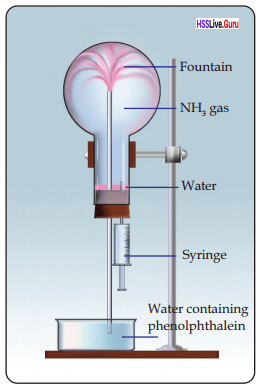What do you observe?
Water in the beaker lifts up in the flask which is filled with ammonia.

Chemistry Chapter 5 Class 10 Kerala Syllabus Question 8.
What inference can be made about the solubility of ammonia in water? Why does water rush into the flask?
Ammonia dissolves abundantly in water. The pressure in the flask decreases with more ammonia dissolves in a water. So more quantity of water lifts up in the flask.

Compounds Of Nonmetals Class 10 Kerala Syllabus  Question 9.
Why does water entering the flask change its color?
The ammonium hydroxide formed by dissolving ammonia in water is an alkali.

Compounds Of Non Metals Class 10 Notes Kerala Syllabus Question 10.
Which property of ammonia is responsible for this change in color?
The property of base/alkali

Kerala Syllabus 10th Standard Chemistry Chapter 5 Question 11.
Complete the chemical equation given below and find the product obtained when ammonia is dissolved in water.
NH3 + H2O → NH2OH

Sslc Chemistry Chapter 5 Notes Pdf Kerala Syllabus Question 12.
Tick ✓ which is applicable to ammonia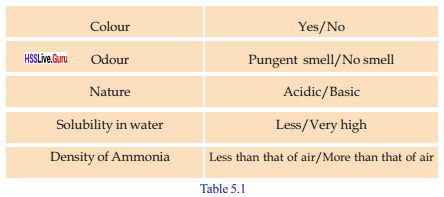Colour Yes/No colour Smell Pungent smell / No smell Property Basic / Acidic Solubility in water More soluble /Less soluble Density Less than air / More than air

Compounds Of Non Metals Kerala Syllabus 10th Standard Question 13.
When an Ammonia tanker leaks, water is sprayed to reduce its intensity. What is the reason for this?
Ammonia dissolves abundantly in water. Ammonia gas is released when the tanker leaks, it dissolves in water and can minimize the spreading of gas into atmosphere.

Compounds Of Non-Metals Class 10 Kerala Syllabus Ques. 14.
Some uses of ammonia are given below.

• For the manufacture of chemical fertilizers like ammonium sulfate, ammonium phosphate, urea, etc.
• As a refrigerant in ice plants.
• To clean tiles and window panes.
• Ammonia is also used as a refrigerant gas, for purification of water sup¬plies, and in the manufacture of plastics, explosives, textiles, pesticides, dyes and other chemicals.
• It is found in many household and industrial-strength cleaning solutions.

Text Book Page No: 82

Sslc Chemistry Chapter 5 Pdf Kerala Syllabus Question 15.
Take some ammonium chloride (NH4Cl) in a boiling tube and heat it. Don’t you sense a peculiar smell?
Yes. Pungent smell

Kerala Syllabus 10th Chemistry Chapter 5 Kerala Syllabus Question 16.
Which is the gas evolved here?
Ammonia.

Hsslive Guru Chemistry 10 Kerala Syllabus Question 17.
Show a wet red litmus paper on the mouth of the boiling tube. What change can you observe?
Turns blue

10th Class Chemistry Chapter 5 Kerala Syllabus Question 18.
Keep the litmus paper for some more time at the mouth of the boiling tube and then observe its color change. What is the change occurred?
The wet litmus paper has changed again to red color due to the presence of the hydrogen chloride (HCl) gas. When ammonium chloride (NH4Cl) is heated. Lighter NH3 comes out first then the denser HCl comes out.

Class 10 Chemistry Kerala Syllabus Question 19.
Write the chemical equation of this reaction.
NH4Cl(S) → NH3(g) + HCl (g)

Text Book Page No: 83

Question 20.
A glass rod dipped in cone. HCl is, shown into the jar which is filled with ammonia. What do you observe?
White smoke is formed.

Question 21.
Complete the equation and find out the product?
NH3 + HCl → NH4C1
NH4Cl is the product. The formation of NH4Cl is the reason for the white smoke.

Question 22.
What happens to the white powder on heating?
It disappears

Text Book Page No: 84

Question 23.
Examine the chemical equations given below and write the forward and backward reactions in each.
1. N2 (g) + 3H2(g) $$\rightleftharpoons$$ 2NH3(g)
2. 2SO2 (g)+ O2 (g) $$\rightleftharpoons$$ 2SO3(g)
3. H2(g) + I2 (g) $$\rightleftharpoons$$ 2HI (g)
1. N2 (g) + 3H2(g) $$\rightleftharpoons$$ 2NH3(g)
Forward reaction:
N2 (g) + 3H2(g) → 2NH3(g) 1
Backward reaction:
2NH3(g) → N2 (g) + 3H2(g)

2. 2SO2 (g)+ O2 (g) $$\rightleftharpoons$$ 2SO3(g)
Forward reaction:
2SO2 (g)+ O2 (g) → 2SO3(g)
Backward reaction:
2SO3(g) → 2SO2 (g)+ O2 (g)

3. H2(g) + I2 (g) $$\rightleftharpoons$$ 2HI (g)
Forward reaction:
H2(g) + I2 (g) → 2HI (g)
Backward reaction:
2HI (g) → H2(g) + I2(g)

Text Book Page No: 85

Question 24.
What happens to the rates of forward and backward reactions as time progresses?
As the time passes, rate of forward reaction decreases and rate of backward reaction increases.

Question 25.
Identify the point at which the rates of both forward and backward reactions become equal?
A

Text Book Page No: 86

Question 26.
The rate of which reaction increases when the concentration of nitrogen is increased? Forward reaction/ Backward reaction.
Forward reaction

Question 27.
What happens if the concentration of ammonia is increased?
Rate of backward reaction increases

Question 28.
What will be the effect of removing ammonia continuously from the system?
Rate of forward reaction increases.

Question 29.
Complete the table writing the effect of change in concentration in the system at equilibrium.Action Change of concentration Change in rate More hydrogen is added Increase the concentration of reactant Rate of forward reaction increases More ammonia is added Increases the concentration of the product Rate of backward reaction increases Ammonia is removed Decreases the concentration of the product Rate of forward reaction increases More nitrogen is added Increases the concentration of the reactant Rate of forward reaction increases

Question 30.
N2(g) + 3H2(g) $$\rightleftharpoons$$ 2NH3(g)
In this equation what is the total number of moles of the reactant molecules?
Total number of moles of the reactant = 4

Text Book Page No: 87

Question 31.
2

Question 32.
Forward reaction : 4 mole reactant molecule → 2 mole product molecules ( volume decreases)
Backward reaction : …. (a) …..mole product molecules → ….(b) ….. mole reactant molecules (volume ……(c) …….)
a. 2
b. 4
c. increases

Question 33.
In the manufacture of ammonia, the reaction in which direction results in the decrease in the number of molecules?
Forward reaction.

Question 34.
What happens when the pressure of system is decreased?
Rate of forward reaction increases.

Question 35.
What if the pressure of the system is decreased?
Rate of backward reaction increases.

Question 36.
In the manufacture of ammonia, why is a pressure of 150-300 atm used?
When pressure is increased, rate of forward reaction increases. As a result, there will be an increase in the yield of product.

Question 37.
H2(g) + I2(g) $$\rightleftharpoons$$ 2HI(g)
What is the total number of moles of reactants?
2

Question 38.
2

Text Book Page No: 88

Question 39.
N2 (g) + 3H2 (g) – 2NH3(g) + Heat
Which is the endothermic reaction in this ?
Forward reaction / Backward reaction
Backward reaction.

Text Book Page No: 89

Question 40.
Sulphuric acid is formed also by the direct dissolution of sulfur trioxide in water. Still, sulfur trioxide is not directly dissolved in water. Why?
The dissolution of sulfur trioxide in water is an exothermic process. It may turn sulphuric acid initially formed into fine fog-like particles (smog) which will hinder further dissolution.

Text Book Page No: 90

Question 41.
Complete the flow chart.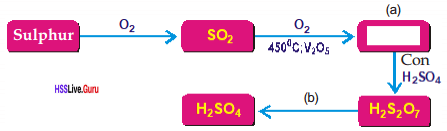a. SO3
b. H2O

Question 42.
Take 5mL water in a test tube and slowly add concentrated sulphuric acid to it. Touch the bottom of the test tube. What do you feel? Is the reaction exothermic or endothermic?
Feels hot. It is a exothermic reaction.

Text Book Page No: 91

Question 43.
What are the constituent elements of sugar?
Carbon, Hydrogen, and Oxygen

Question 44.
Which is the black substance in the product formed?
Carbon

Question 45.
What is the ratio of hydrogen and oxygen in sugar?
2: 1

Question 46.
Which is the substance that absorbed hydrogen and oxygen from sugar in the ratio as in water?
Conc. H2SO4

Question 47.
Complete the table by involving the activities given below.No. Activity Observation 1 Dropping Con. H2SO4 on a cotton cloth. Clothes will get burnt. It will extract water out of your clothes and all will be left will be carbon alongside some other things. 2 Adding Con. H2SO4 to glucose taken in a small beaker. The sulfuric acid removes water from the sugar in a highly exother­mic reaction, releasing heat, steam, and sulfur oxide fumes. The white sugar turns into a black carbonized tube that pushes itself out of the beaker 3 Adding Con. H2SO4 to a watch glass in which CuSO4 crystals are taken. Blue copper sulfate crystals it was decolorised. Sulphuric acid is an strong dehydrating acid therefore, it removes Oxygen.

Question 48.
Why is concentrated sulphuric acid not used as a drying agent in the preparation of ammonia?
Ammonia is a base. Ammonia reacts with sulphuric acid and the salt ammonium sulfate is produced.
2NH3 + H2SO4 → (NH2)2SO4

Text Book Page No: 92

Question 49.
Add concentrated sulphuric acid to a test tube containing a small quantity of carbon. Heat it. What do you observe?
Gases are formed. CO2 and SO2 are the gases.

Question 50.
Analyze the chemical equation and find the reason for your observation.a. What is the oxidation state of elemental carbon?
Zero

b. What about the carbon in carbon dioxide?
The oxidation state of carbon in carbon dioxide is +4.

c. Was carbon oxidized or reduced in this reaction?
Carbon is oxidized.

d. Which is the oxidizing agent?
Sulphuric acid is the oxidizing agent.

Question 51.
See the reaction between concentrated sulphuric acid and copper.a. Is copper oxidized or reduced in this case?
Copper is oxidized.

b. Which is the oxidizing agent in this reaction? Which is the reducing agent?
Copper is the reducing agent.
Sulphuric acid is the oxidizing agent.

Text Book Page No: 93

Question 52.
Analyze the given chemical equation.
Na2SO4 + BaCl2 → BaSO4 + 2NaCl

a. Which substance is soluble in water among the products?
NaCl

b. Which substance is the white precipitate?
Barium sulphate (BaSO4).

c. Does the white precipitate dis-solve when dilute hydrochloric acid is added to it?
No. It is not soluble in dilute HCl

Question 53.
Write down the observation in the table given below, when lmL Barium chloride solution is added to the solutions given in the table.No. Solution By adding BaCh solution When dilute HCl is added to this 1. MgSO4 Barium sulfate (BaSO4) formed. A white precipitate. Insoluble in dil HCl. Precipitate not disappear. 2. ZnSO4 Barium sulfate (BaSO4) formed. A white precipitate. Insoluble in dil HCl. Precipitate not disappear.

Let Us Assess

Question 1.
In which of the following reversible reactions does change in pressure not influence equilibrium? What is the reason?
i. H2(g) + I2(g) $$\rightleftharpoons$$ 2HI
ii. N2 + 3H2(g) $$\rightleftharpoons$$ 2NH3
i. H2(g) + I2(g) $$\rightleftharpoons$$ 2 Hg(g)
In this reaction, the number of moles of the reactants and the number of moles of the products are same.

Question 2.
What is the use of applying high pressure during the formation of ammonia from nitrogen and hydrogen?
In this reaction, the number of moles of the products is less than the number of moles of the reactants. So if the pressure is increased, rate of forward reaction is increased. Thereby the yield of the product will be more.

Question 3.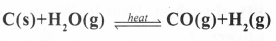a. Identify the reactants and products.
b. Products are frequently removed from the system? Explain the reason.
a. Reactants : C(s), H2O(g)
Products: CO(g), H2(g)
b. When the products are frequently re moved from the system, rate of forward reaction increases. Hence more products will be formed.

Question 4.
2NO(g) + O2(g) $$\rightleftharpoons$$ 2NO2(g) + heat
In this reaction how do the following changes influence the amount of the product?
a. Decrease in temperature
b. Increase in pressure
c. Increase in concentration of oxygen
a. When the temperature is decreased, rate of forward reaction increases. Hence more products is formed.
b. When pressure is increased, rate of forward reaction increases. Hence more product is formed.
c. When the concentration of oxygen is increased the rate of forward reaction increases. Hence more product is formed.

Question 5.
N2(g) + 3H2(g) $$\rightleftharpoons$$ 2NH3(g) + heat
a. What change in pressure is required for the maximum yield of the product?
b. What is the change in concentration required for increasing the rate of the forward reaction?
a. On increasing the pressure, yield of the product will be maximum.
b. Increase the concentration of reactants nitrogen or hydrogen. Removal of product ammonia from the system.

Question 6.
The chemical equation of one of the different stages of manufacturing sulphuric acid by contact process is given below. Find out the influence of the following factors in the reaction given below.
2SO2 (g) + O2 (g) $$\rightleftharpoons$$ 2SO3(g) + heat
1. Increase the amount of oxygen
2. Pressure is increased
4. SO3 is removed
1. Rate of forward reaction increases.
2. Rate of forward reaction increases
3. Attains the equilibrium quickly.
4. Rate of forward reaction increases

Question 7.
Calcium oxide (CaO) is used as drying agent in the preparation of Ammonia in laboratory. Can concentrated H2SO4 be used as drying agent instead of CaO? Justify your answer.
Sulphuric acid can not be used instead of CaO. it is because sulphuric acid reacts with ammonia and the salt ammonium sulfate is formed.

Question 8.
Which property of sulphuric acid is shown in the following situations.
a. During the preparation of chlorine, the gas is passed through concentrated H2SO4
b. Wooden cupboards appeared to be burnt when concentrated sulphuric acid happened to fall on it.
a. It’s character as a drying agent
b. It’s character as a dehydrating agent.

Extended Activities

Question 1.
The graph for the reaction N2(g) + 3H2(g) $$\rightleftharpoons$$ 2NH3(g)+ heat is given below.a. Identify and write the reactions C and D
b. What happens to the position of point A in the graph when a catalyst is used? Redraw the graph.
a Reaction C – Forward reaction,
Reaction D – Backward reaction,
b. Equilibrium is attained quickly. Point A shift to the left side.Question 2.
It is often said that the production of sulphuric acid is a bench mark of the industrial development of a country. Prepare a note based on the various uses of sulphuric acid.
Sulphuric acid is an important industrial chemical which is used in the manufacturing processes of many goods over a wide range of applications. Sulfuric acid used in pulp and paper industry for chlorine dioxide generation, tall oil splitting and pH-adjustments.Sulfuric acid is a strongly acidic, oily liquid that may be clear to cloudy in appearance. Concentrated sulfuric acid acts as both an oxidizing and dehydrating agent.

Sulfuric acid was once known as oil of vitriol. Here are some of the growing number of end-users and applications using sulfuric acid is Agricultural chemicals Aluminum Sulfate, Batteries, Cellophane, Detergents, Explosives, Fertilizers, Gasoline, Herbicides, Iron and steel pickling, Jet Fuel, Kerosene, Leather, Lubricating Oils, Medicinal processes, Oil additives, Paper, Rayon and rubber, Sugar, Synthetic fibers, Veterinary drugs, Water softener regeneration, Water treatment, Yellow pigments.

Question 3.
Fill half of a beaker of capacity 50 mL with sugar. Add concentrated sulphuric acid so that the sugar is immersed in it. Observe the changes. What are the products formed? Which property of sulphuric acid is revealed here?
The sulphuric acid removes water from the sugar in a highly exothermic reaction, releasing heat, steam, and sulfur oxide fumes. Aside from the sulfurous odor, the reaction smells a lot like caramel. The white sugar turns into a black carbonized tube that pushes itself out of the beaker. Dehydrating properties of H2SO4 are shown in the experiment. Concentrated sulphuric acid has the ability to absorb chemically combined water, or hydrogen and oxygen from substances in the ratio corresponding to that of water. This process is known as dehydration. Concentrated sulphuric acid is a strong dehydrating agent.

### Compounds of Non-Metals Orukkam Questions and Answers

Question 1.
a. Find out the relation between Temperature and rate of a reaction?
Select the materials from the list above
Sodium the Sulphate, Test tube, dil HCl, Cu, Mg ribbon, Beaker, Water, Spirit lamp, boding Tube
b. Prepare a write up for finding the relation between temperature and rate of a reaction?
c. Why rate of a reaction increase when temperature increases?
a. Essential Materials:- Sodium Thiosu- phate, Hydrochloric Acid, Water, Boiling Tube, Spirit Lamp. Prepare a solution of diluted sodium Thiosulphate in a beaker. Take equal amount of this solution in two Boiling Tubes. Heat one of the boiling tubes for some time. Add equal amount of diluted HCl to both the boiling tubes.
b. The Essential material/Materials Required Mg Ribbon, Cone HCl, dil HCl
Write up
Take Magnesium Ribbons of equal mass in two test tubes. Add dil HC1 to one test tube and dilute HC1 to the other in equal volumes.
c. Energy as well as the speed of molecules increases when reactants are heated. That is as temperature increases the number of molecules with three hold energy increases. As a result, the number of effective collisions increases and these rate of reaction also increases.

Question 2.
Some chemical reactions are given below.
1. Zn + 2HCI → ZnCl2 + H2
2. 2Mg + O2 → 2MgO
3. NH4Cl $$\rightleftharpoons$$ NH3 +HCl
a. What are the peculiarities of first two reactions
b. Conduct an experiment for viewing the dissociation and association taking place in the third equation.
c. In the three reactions, reactants turned into product and products are converted into reactants, is it true? d What type of reactions are they all represent?
e. Write down the characteristics of the re-action.
a. In these reactions, the reactants change to products but the products cannot be changed back to reactants.
b. Heat some Ammonium chloride in a boiling tube.
c. No
d. First two Reactions are irreversible reactions. Third Reaction is a reversible Reaction.
e. Characteristics of Irreversible Reaction:- Reactants become products but products cannot be changed back to Reactants.
Characteristics of Reversible Reaction:- Reaction takes place in both directions.

Question 3.
Fe(NO3)3 + 3KCNS → Fe(CNS) + 3KNO3 This balanced chemical equation is wrote on the black board when the teacher is going to conduct an experiment on chemical equilibrium.
a. In the above reaction, which chemical has red color.
b. Fe(NO3)3, KCNS combined together, put it on the test tube stand, is there any col our change? Is the color is diminishing while it kept in test tube stand?
c. Convert the solution reared into four be a kers dilute each with equal amount of water.
In the first beaker add Fe (NO3)3, in the second one KCNS, in the third KNO4 like wise. Compare the color change with the fourth beaker. Find out the reason.
d. Point out the characteristics of equilibrium based on the experiment done.
e. In minute level chemical equilibrium is Kinetic energy why?
f. How and when a reversible reaction attains chemical equilibrium.
g. In the graph given below, when the reactant and product attain the level A? What are the characteristics of the point A?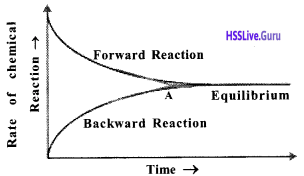a. Fe (CNS), (Ferric Thiocyanate)
b. Yes, Red color increases
c.

 Activity Performed Observations Reason Added Fe (NO3)3 Red color of solution inten­sifies Fe (NO3)3 reacted with the remaining KCNS to form the product Added KCNS The red color of solution intensifies KCNS reacted with remain­ing in the solution to form more product Added KNO3 Intensity of the red color of the solution is reduced significantly The product Fe (CNS), is reacted with KNO3 to form reactants.

d. At equilibrium both the reactants and products coexist.

• The rates of forward and backward reactions become equal at equilibrium.
• Chemical equilibrium is dynamic at the molecular level.
• The system can attain equilibrium even when the concentration of the reactants and products are not equal. Once equilibrium has been attained, there will be no change in the concentration of reactants as well as that of products.
• Chemical equilibrium is attained in closed system.

e. Even in equilibrium state reactants, molecules form products and products form reactants. Hence in minute level chemical equilibrium is kinetic energy.
f. The concentration of reactants and products do not change a reversible reaction attain chemical equilibrium,
g. A is the point at which the rates of both forward and backward reactions become equal.
At equilibrium both the reactants and products coexist.

Question 4.
N2 + 3H2 $$\rightleftharpoons$$ 2NH3
H2+I2 $$\rightleftharpoons$$ 2Hl
N2O4(g) $$\rightleftharpoons$$ 2NO3
2SO2 + O2 $$\rightleftharpoons$$ 2SO3
Write down detail how amount of the prod¬ucts increases in the above reactions (based on Le chandelier principle)
Hints: reference must be given on each of the following, concentration, pressure, temperature, catalyst

• Increase the concentration of reactants or anyone reactants.
• Increase the temperature – Increases the rate of endothermic reactions.
• Decrease the temperature- Increase the rate of exothermic reactions. Increase the pressure- Reaction takes place faster in the direction where the molecules are less.
• Decrease the pressure- Reaction takes place faster in the direction where molecules are more.
• Catalysts are added at the beginning of a chemical reaction.

### Compounds of Non-Metals SCERT Questions and Answers

Question 5.
The graph showing the progress of the reaction N2 + 3H2 $$\rightleftharpoons$$ 2NH3 is given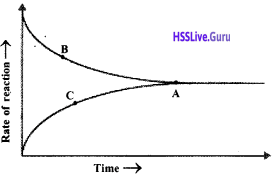a. Identify the reactions represented by B and C?
b. What is the significance of the state A?
c. Is there any change in the concentration, as time passes after attaining the stage A? Explain.
a. B – Forward reaction, C – Backward reaction
b. Equilibrium
c. No At equilibrium rate of both forward and backward reactions are equal.

Question 6.
Coldwater is taken in one test tube an hot water in another one. Mg ribbon with same size is dropped in each of the test tubes,
a. In which test tube, hydrogen is formed with greater speed?
b. Which factor influences the rate of reaction? Explain the reason.
a. Test Tube which contains hot water.
b. Temperature, When temperature increases the number of molecules with thresh-old energy increases. As a result number of effective collisions increases.

Question 7.
The chemical equation of the industrial preparation of ammonia is given below.
N2 + 3H2 $$\rightleftharpoons$$ 2NH3 + Heat
Suggest the methods to get more NH3.
Increase the Pressure and Temperature.

Question 8.
H2(g) + I2(g) $$\rightleftharpoons$$ 2HI(g) + Heat
The following circumstances influence the reaction
a. Increase the concentration of H2.
b. Increase the pressure
c. Increase the temperature.
a. Increases the rate of forward reaction
b. No effect
c. Increases the rate of backward reaction

Question 9.
The formation of SO3 in the industrial preparation of sulphuric acid is given below.
2SO2 + O2 $$\rightleftharpoons$$ 2SO3+ Heat
a. Explain the effect of concentration of 02 to get maximum yield of SO3? State rea son.
b. Identify the law related to it. State it.
a. Increase the concentration of oxygen, which increases the rate of forward reaction,
b. When the concentration, pressure or tern perature of a system at equilibrium is changed, the system will readjust itself so as to nullify the effect of that change and attain a new state of equilibrium. This is Le Chatelier’s principle.

Question 10.
The chemical equation of a stage in the industrial preparation of sulphuric acid is given below.
2SO2 + O2 $$\rightleftharpoons$$ 2SO3+ Heat
a. Which is the catalyst used in this reaction?
b. What is the influence of the catalyst in equilibrium?
b. Catalyst increases the rate of both the forward and backward reactions to the same extent.

Question 11.
The chemical equation of the industrial preparation of ammonia is given below.
N2 + 3H2 $$\rightleftharpoons$$ 2NH3+ Heat
a. Temperature is to be decreased to get maximum yield of ammonia, according to the Le Chatelier principle. Why?
b. What is the reason for taking an optimum temperature in this reaction?
a. When the temperature decreases exothermic reaction increases ie. forward reaction increases.
b. At low-temperature rate of forward and backward reaction is slow.

Question 12.
Analyse the following equations and answer the questionsa. Which of these reactions are affected by change in pressure? What are the reasons?
b. How the increase in pressure influence the reaction which you have already identified ?
a. iii. N2 + 3H2 $$\rightleftharpoons$$ 2NH3
In first reaction NH4Cl is solid compound In second reaction number of reactants and product molecules are equal.
b. When pressure increases, rate of forward reaction increases.

Question 13.
Catalysts are substances which influence the rate of chemical reactions.
Explain how the catalysts influence the rate of reversible reaction?
The catalyst increases the rate of both the forward and the backward reactions to the same extent. As a result, the system reaches equilibrium at a faster rate.

Question 14.
Some features of a reversible reaction are given below.
1. Product formation increases when the temperature is increased.
2. There is no effect when the pressure is increased.
Explain the reason for above inferences.
1. Forward reaction is an exothermic.
2. Number of reactant and product molecules

Question 15.
A + B + Heat $$\rightleftharpoons$$ 2C + D
This reversible reaction is in equilibrium. What happens to the amount of products under the following conditions?
a. C is removed from the system
b. B is added in excess
c. Temperature is increased
d. A suitable catalyst is added.
a. Increase the amount of product
b. Increase the amount of product
c. Decrease the amount of product,
d. Increase the amount of product

### Compounds of Non-Metals Exam Oriented Questions and Answers

Very Short Answer Type Questions (Score 1)

Question 16.
Select the correct statements which are related to the influence of catalyst in a reversible reaction.
a. Forward reaction takes place when a cata lyst is used in a reversible reaction, h, Attains equilibrium faster,
c. Catalyst does not help to form more pro-duct
d The catalyst increases the rates of both the forward and the backward reactions to the same extent.
e. Increases the speed of backward reaction.
f. Does not helps to produce more product
Statements b, c, d are correct.

Question 17.
2X(g) + heat $$\rightleftharpoons$$ Y(g) + Z(g) Explain the influence of pressure and temperature in this reaction.
Pressure — Pressure has no effect here. Because the number of molecules in the reactants and products side are equal.
Temperature — Here the forward reaction is endothermic. So increasing temperature increases the rate of forward reaction. Decreasing temperature decreases the rate of forward reaction.

Short Answer Type Questions (Score 2)

Question 18.
Observe the equation of the chemical reactions given below.
i. NaOH (aq) + HCl (aq) → 2NaCl (aq) + H2O(l)
ii. N2(g) + O2(g) $$\rightleftharpoons$$ 2NO(g)
iii. Zn(s) + ZHCl → ZnCl2(ql) + H2(g)
iv. 2SO2(g) + O2(g) $$\rightleftharpoons$$ 2SO3(g)
a In which chemical reaction pressure can influence its speed ?
b. In this reaction what changes will be made in pressure for increasing forward reaction ? Why ?
Reaction (iv)
2SO3(g) + O3(g) $$\rightleftharpoons$$ 2SO3(g)
b. In this reaction, the number of moles of the products is less than the number of moles of the reactants. So if the pressure is increased, rate of forward reaction is increased. Thereby the yield of the product will be more.

Question 19.
A graph given below deals with the reversible reaction.a What does A, B, C indicate?
b. What inference can be drawn about the concentration of the reactants and products at the point D and E?
A – Forward reaction
B – Backward reaction
C – Equilibrium
b. There is no change in the concentration, of reactants and products at the points D and E. Because at the point ‘C’ the process attains equilibrium.

Question 20.
Some chemicals are given below. Sodium chloride, Ammonium hydroxide, Nitric acid, cone. Sulphuric, Sodium hydroxide.
a. Which are the substances needed to produce hydrogen chloride?
b. Suggest a method to identify hydrogen chloride gas.
a. Sodium chloride
b. Show a glass rod dipped in ammonia, just above the jar in which hydrogen chloride gas is taken. A white smoke of NH34Cl is produced.

Question 21.
When ammonia was leaked two solutions were arised.
i) Spray water
ii) Spray HCl
Spray water. Ammonia dissolves abundantly in water. So we can prevent the easy spreading of ammonia. When HCl is used the white smoke of NH4Cl spread in the atmosphere.

Short Answer Type Questions (Score 3)

Question 22.
N2(g) + 3H2(g) $$\rightleftharpoons$$ 2NH3 (g) + heat
How do the following factors influence the forward reaction?
a. One of the products is removed
b. Increase in pressure
a. Speed of forward reaction increases
b. Speed of forward reaction increases
c. Speed of forward reaction increases

Question 23.
A graph given below deals with the reversible reaction.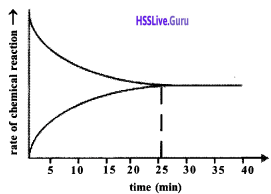a. What happened to the forward and backward reactions as time passes?
b. In which minute does the system attain equilibrium?
c. What change occurs to the equilibrium, when a catalyst is used?
a. As time passes the speed of forward reaction decreases and backward reaction increases.
b. 25th-minute
c. Attain equilibrium fast ie. before 25th minute.

Question 24.
Ammonia is an industrially useful compound of nitrogen.
a. Name the industrial production of ammonia
b. Write the equation of the reaction,
c. Write any two uses of ammonia
a. Haber process
b. N2 + 3H2 → 2NH3
c. i. For the manufacture of the fertilizers
ii. As a coolant in ice plants.

Question 25.
Ammonia is a pungent-smelling gas.
a. How does ammonia convert into
i. liquor ammonia
ii. liquid ammonia
b. Write the color, smell, solubility in water and density of ammonia.
a. i. Prepare a concentrated solution of ammonia by dissolving it in water. It is liquor ammonia.
ii. The gaseous ammonia can be converted into liquid by applying pressure. It is called liquid ammonia.
b. Ammonia has no color and irritating smell. It dissolves abundantly in water. It has density less than air.

Question 26.
Sulphuric acid is industrially manufactured by using contact process. Write the equation of this process.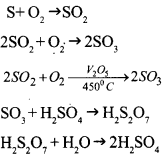Question 27.
Write equations for the following chemicals to prepare from sulphuric acid.
a. Hydrogen chloride
b. Oleum
c. Sodium sulfate
a. NaCl + H2SO4 → NaHSO4 + HCl
b. H2SO4 + SO3 → H2S2O7
c. 2NaOH + H2SO4 → Na2SO4 + 2H2O

Question 28.
Observe the following chemical equation
Cu + 2H2SO4 → CuSO4 + SO2 + 2H2O
a Which one has oxidation state as 0?
b. What is its oxidation state after the chemical reaction?
c. Is the change oxidation or reduction?
d. Which chemical nature of sulphuric acid is found here?
a. Cu
b. 2+
c. Oxidation
d. Nature of oxidation

Question 29.
Litmus has colored in the solution.
A. When BaCl2 solution was added to this.
B. Solution, a white precipitate insoluble in HCl is produced.
1. Write the chemical formula of A.
2. Write the chemical name and chemical formula of the white precipitate produced when it was added with BaCl2.
c. Complete the equation
C + 2(A) →…….. + …… +……..
a. H2SO4
b. Barium sulphate (BaSO4)
c. C + 2H2SO4 → CO2 + 2H2O + SO2

Question 30.
Analyze the table given below and complete it.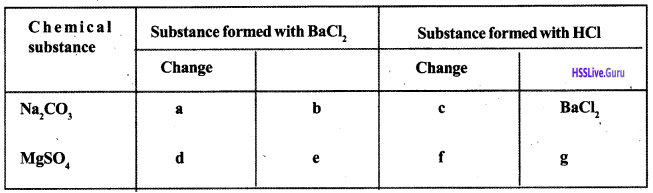a. White precipitate is formed
b. Ba2CO3
c. Clear solution
d. White precipitate is formed
e. BaSO4
f. No change
g. No substance is formed

Question 31.
Sulphuric acid is a drying agent as well as a dehydrating agent.
a. Find out the difference between these two activities.
b. Write one example each for these nature of sulphuric acid.
a. Dehydration is the process of absorbing hydrogen and oxygen from substances in the ratio of water.
Drying activity is the process of absorbing water content present in a substance.
b. Sugar bums to black when concentrated sulphuric acid is added in it. It is an example for dehydration.
Concentrated H2SO4 is used to remove water particles from HCl gas during its manufacture – drying agent.

Question 32.
Some incomplete equations are given below:
i. MgSO4 + BaCl2 → …….. + MgCl2
ii. K2CO3 + BaCl2 → ……… + 2KC1
iii. KCl + AgNO3 → …….. + KNO3
a. Complete the equations.
b. Find out the white precipitate in each of the reactions.